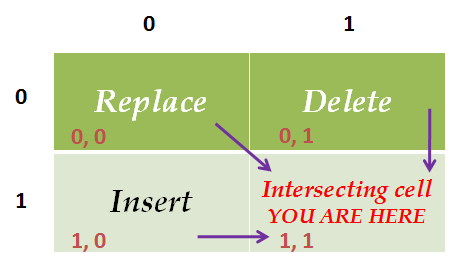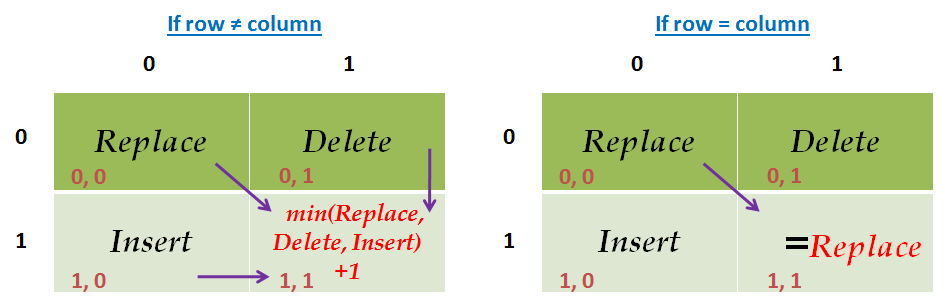Advanced Database Management System - Tutorials and Notes: Calculate minimum edit distance between strings using Levenshtein distance

## Find minimum edit distance between two strings with Levenshtein distance

Question:

Find the minimum edit distance in transforming the word DOG to COW using Levenshtein distance, ie., insertion = deletion =1 and substitution = 2.

Solution:

### Minimum edit distance algorithm finds the minimum number of editing operations (insertion, deletion, substitution) required to convert one string into another with the help of dynamic programming concept.

In this exercise, we supposed to use Levenshtein distance while finding the distance between the words DOG and COW. Each cell in the distance matrix contains the distance between two strings. For instance, the cell intersect at i, j (distance[i, j]) contains the distance between first i characters of the target and the first j characters of the source. The value for each cell is calculated as per the equation shown below for Levenshtein distance;

Step-by-step process is as follows;
Step 1: Draw the edit distance matrix. In this, each word is preceded by # symbol which marks the empty string.
 # C O W # D O G

Step 2: Find the edit-distance values using minimum edit distance algorithm to convert # (row side) to #COW (column side) and populate appropriate cells with the calculated distance.
Number of operations required to convert;
·        # to # is 0.
·        # to #C is 1. That is, one insertion (no change with #, insert C)
·        # to #CO is 2. That is, two insertions (no change with #, insert C and O)
·        # to #COW is 3. That is, three insertions (no change with #, insert C, O and W)
 # C O W # 0 1 2 3 D O G

Step 3: Find the edit-distance values using minimum edit distance algorithm to convert # (column side) to #DOG (row side) and populate appropriate cells with the calculated distance.
Number of operations required to convert
·        # to # is 0.
·        # to #D is 1. [one insertion (no change with #, insert D]
·        # to #DO is 2. [two insertions (no change #, insert D and O]
·        # to #DOG is 3. [three insertions (no change #, insert D, O and G]
 # C O W # 0 1 2 3 D 1 O 2 G 3

So far, we have found the minimum edit distance for 7 sub-problems.
[# - # = 0, # - #C = 1, # - #CO = 2, # - #COW = 3, # - #D = 1, # - #DO = 2, and # - #DOG = 3]

Step 4: From this step onward, we try to find the cost for a sub-problem by finding the minimum cost of three sub-problems and add 1 with that if the characters intersect at that cell are different. If the intersecting characters are same, then we add 0 with the diagonal cell value.
[Note: we have used A as the name for this matrix and included the index numbers for easy understanding]
Use the following table as a key to find the cost.If the row character ≠ column character,
The cost of the intersecting cell = min(replace, delete, insert)+2.
If the row character = column character,
The cost of the intersecting cell = cost of the Replace cellSub-problem: #D à #C.
Intersecting cell: A[1, 1]
Same intersecting characters? NO.
Cost(A[1, 1])       = Min(A[0, 0], A[0, 1], A[1, 0]) + 2
= A[0, 0] + 2
= 0 + 2 = 2
 A 0 1 2 3 # C O W 0 # 0 1 2 3 1 D 1 2 2 O 2 3 G 3

Sub-problem: #D à #CO.
Intersecting cell: A[1, 2]
Same intersecting characters? NO.
Cost(A[1, 2])       = Min(A[0, 1], A[0, 2], A[1, 1]) + 2
= A[0, 1] + 2
= 1 + 2 = 3
 A 0 1 2 3 # C O W 0 # 0 1 2 3 1 D 1 2 3 2 O 2 3 G 3

Sub-problem: #D à #COW.
Intersecting cell: A[1, 3]
Same intersecting characters? NO.
Cost(A[1, 3])       = Min(A[0, 2], A[0, 3], A[1, 2]) + 2
= A[0, 2] + 2
= 2 + 2 = 4
 A 0 1 2 3 # C O W 0 # 0 1 2 3 1 D 1 2 3 4 2 O 2 3 G 3

Sub-problem: #DO à #C.
Intersecting cell: A[2, 1]
Same intersecting characters? NO.
Cost(A[2, 1])       = Min(A[1, 0], A[1, 1], A[2, 0]) + 2
= A[1, 0] + 2
= 1 + 2 = 3
 A 0 1 2 3 # C O W 0 # 0 1 2 3 1 D 1 2 3 4 2 O 2 3 3 G 3

Sub-problem: #DO à #CO.
Intersecting cell: A[2, 2]
Same intersecting characters? YES
Cost(A[2, 2])       = Min(A[1, 1], A[1, 2], A[2, 1]) + 0
= A[1, 1] + 0
= 2 + 0 = 2
 A 0 1 2 3 # C O W 0 # 0 1 2 3 1 D 1 2 3 4 2 O 2 3 2 3 G 3

Sub-problem: #DO à #COW.
Intersecting cell: A[2, 3]
Same intersecting characters? NO.
Cost(A[2, 3])       = Min(A[1, 2], A[1, 3], A[2, 2]) + 2
= A[2, 2] + 2
= 2 + 2 = 4
 A 0 1 2 3 # C O W 0 # 0 1 2 3 1 D 1 2 3 4 2 O 2 3 2 4 3 G 3

Sub-problem: #DOG à #C.
Intersecting cell: A[3, 1]
Same intersecting characters? NO.
Cost(A[3, 1])       = Min(A[2, 0], A[2, 1], A[3, 0]) + 2
= A[2, 0] + 2
= 2 + 2 = 4
 A 0 1 2 3 # C O W 0 # 0 1 2 3 1 D 1 2 3 4 2 O 2 3 2 4 3 G 3 4

Sub-problem: #DOG à #CO.
Intersecting cell: A[3, 2]
Same intersecting characters? NO
Cost(A[3, 2])       = Min(A[2, 1], A[2, 2], A[3, 1]) + 2
= A[2, 2] + 2
= 2 + 2 = 4
 A 0 1 2 3 # C O W 0 # 0 1 2 3 1 D 1 2 3 4 2 O 2 3 2 4 3 G 3 4 4

Sub-problem: #DOG à #COW.
Intersecting cell: A[3, 3]
Same intersecting characters? NO.
Cost(A[3, 3])       = Min(A[2, 2], A[2, 3], A[3, 2]) + 2
= A[2, 2] + 2
= 2 + 2 = 4
 A 0 1 2 3 # C O W 0 # 0 1 2 3 1 D 1 2 3 4 2 O 2 3 2 4 3 G 3 4 4 4
Result:

### The distance between two strings DOG and COW as per Minimum Edit Distance using Levenshtein distance is 4.

***********

Go to NLP Glossary